Question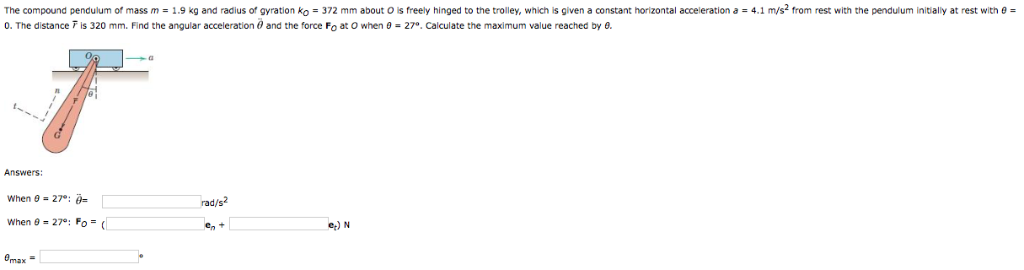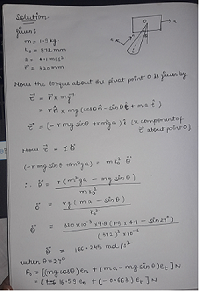#### Earn Coins

Coins can be redeemed for fabulous gifts.

Similar Homework Help Questions
• ### 2. The 100-kg pendulum has a center of mass at G and a radius of gyration...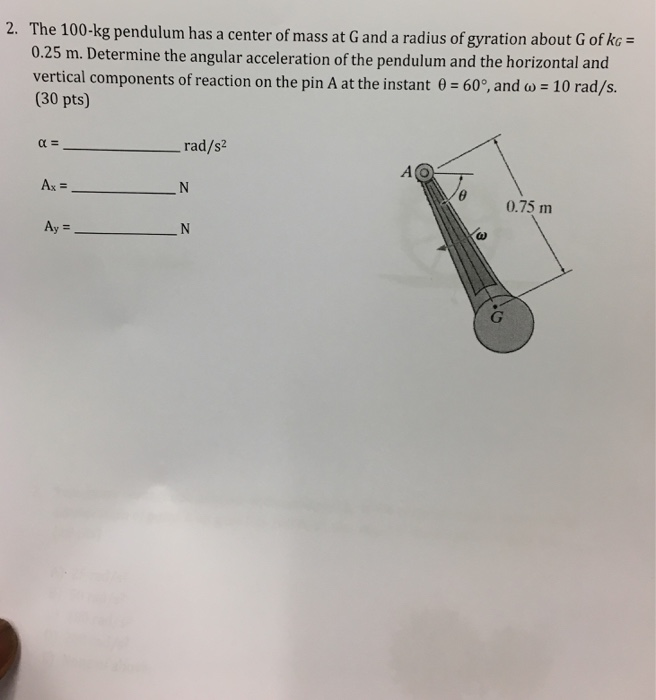2. The 100-kg pendulum has a center of mass at G and a radius of gyration about G of kG 0.25 m. Determine the angular acceleration of the pendulum and the horizontal and vertical components of reaction on the pin A at the instant ?-60°, and ?-10 rad/s. (30 pts) rad/s2 0.75 m

• ### PROBLEM 4 The 30-Kg pendulum has its mass center at G and a radius of gyration about point G of -...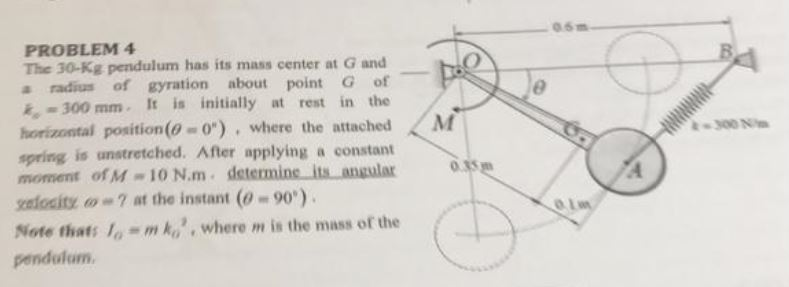PROBLEM 4 The 30-Kg pendulum has its mass center at G and a radius of gyration about point G of - ,#300 mm . It is initially at rest in the horizontal position(6-0") , where the attached <M spring is unstretched. After applying a constant moment ofM " 10 N.m . determine angular 300 N 2nlositz ω" ? at the instant (-90° ) . Note that: -m ko,, where m is the mass or the pendulum PROBLEM 4 The 30-Kg...

• ### Gear Chas a mass of 13 kg and a centroidal radius of gyration of 75 mm. The uniform bar AB has a ...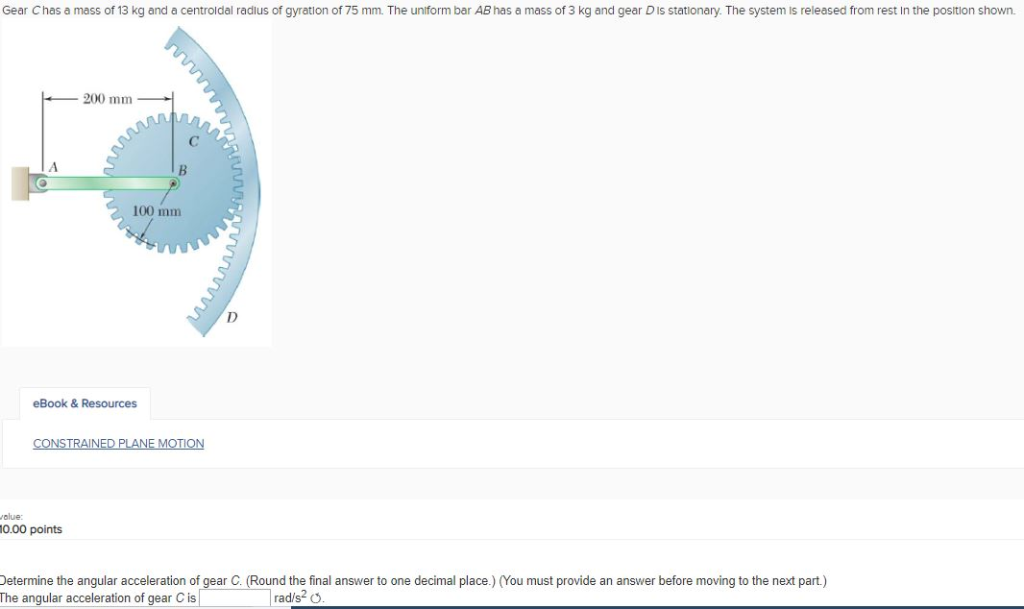Gear Chas a mass of 13 kg and a centroidal radius of gyration of 75 mm. The uniform bar AB has a mass of 3 kg and gear D is stationary. The system is released from rest in the position shown 200 mm 100 mm eBook & Resources olue: 0.00 points Determine the angular acceleration of gear C. Round the final answer to one decimal place.) (You must provide an answer before moving to the next part) The angular acceleration...

• ### The wheel has a mass of 7,074 kg and has a radius or gyration 0 T78 m The outside dius is 1 m and...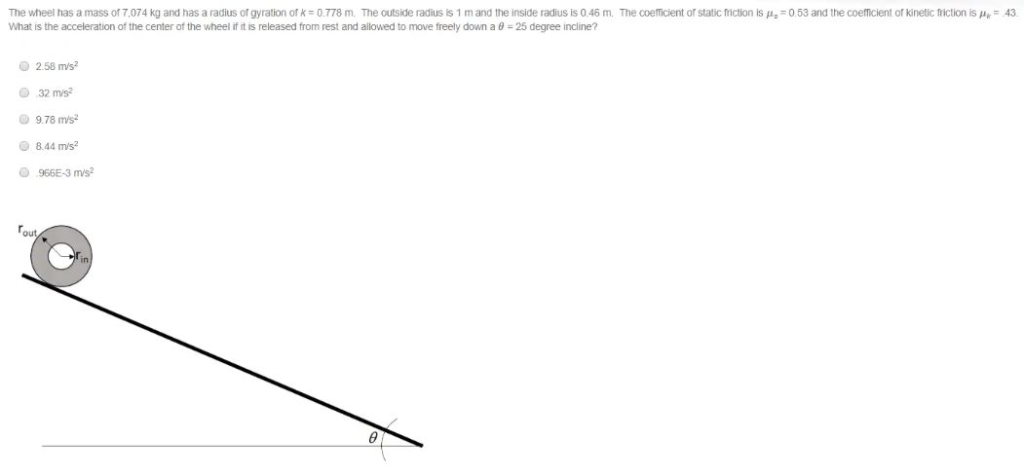The wheel has a mass of 7,074 kg and has a radius or gyration 0 T78 m The outside dius is 1 m and he inside adi is 046m What is the acceleration of the center of the wheel ifit เร released from rest and allowed to move freely down a θ-25 degree incline? The coe cent or state mo ko s μ. 3 and he c emc er t kinetic ict ms 2.58 m/s? O 32 msi 9.78 mis...

• ### 2. The grooved drum has mass m 12 kg, radius of gyration ko-210 mm, groove radius...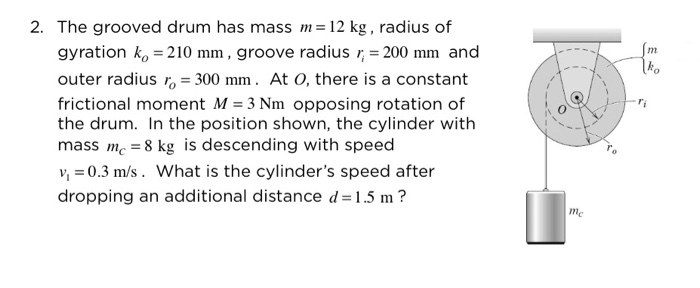2. The grooved drum has mass m 12 kg, radius of gyration ko-210 mm, groove radius r 200 mm and outer radius ro 300 mm. At O, there is a constant frictional moment M 3 Nm opposing rotation of the drum. In the position shown, the cylinder with mass mc 8 kg is descending with speed v0.3 m/s. What is the cylinder's speed after dropping an additional distance d 1.5 m? nm ko me

• ### The 24-kg roll of paper has a radius of gyration ka = 90 mm about an...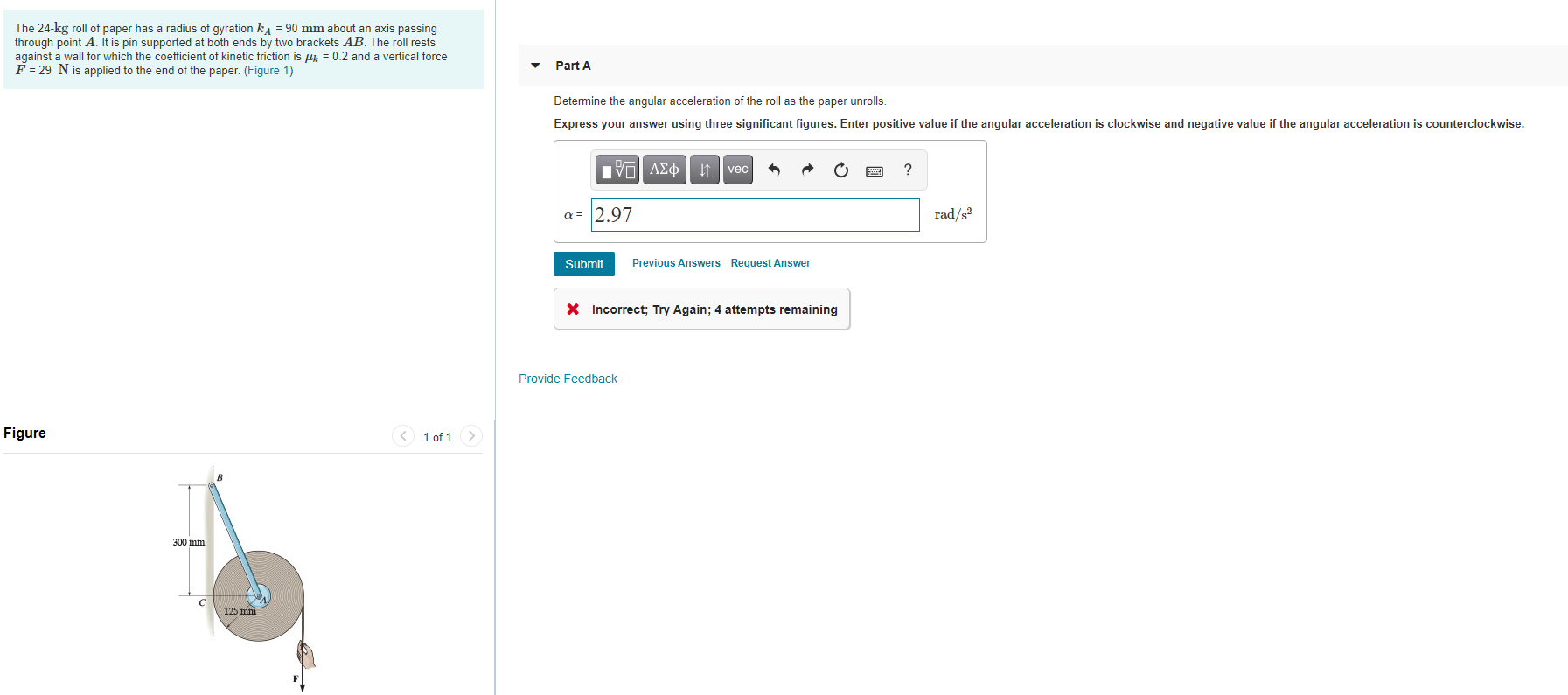The 24-kg roll of paper has a radius of gyration ka = 90 mm about an axis passing through point A. It is pin supported at both ends by two brackets AB. The roll rests against a wall for which the coefficient of kinetic friction is like = 0.2 and a vertical force F = 29 N is applied to the end of the paper. (Figure 1) Part A Determine the angular acceleration of the roll as the paper unrolls...

• ### A 7.5-kg wheel, with a radius of 0.3 m and a radius of gyration about point...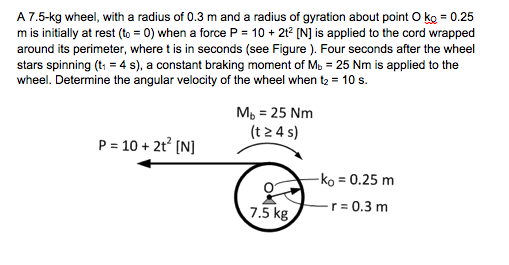A 7.5-kg wheel, with a radius of 0.3 m and a radius of gyration about point O ko 0.25 7.5-kg wheel, with a m is initially at rest (to 0) when a force P 10 2t2 IN] is applied to the cord wrapped around its perimeter, where t is in seconds (see Figure ). Four seconds after the wheel stars spinning (t14 s), a constant braking moment of Mb 25 Nm is applied to the wheel. Determine the angular velocity...

• ### Item 2 C20f6 Tho 1 radius of gyration about G of kg-250 mm. Noglect the weight...Item 2 C20f6 Tho 1 radius of gyration about G of kg-250 mm. Noglect the weight of the beam and the support. At the instant 0- 90° the pendulum is rotating at w 8 rad/s (Figure 1) Figure Request Anwer 0.75 m Determine the magnitude Express your tee nrmal reaction of the roler Bat te instant θ"W' answer to three signiticant figures and include the appropriate units. Units 2r3T4

• ### A 16 N m torque is applied to a wheel of mass 1 kg and radius...

A 16 N m torque is applied to a wheel of mass 1 kg and radius of gyration of 2 m. What is the resulting angular acceleration? a) 3 rad/s2 b) 4 rad/s2 c) 6 rad/s2 d) 10 rad/s2 e) none of the above

• ### 2. As shown below a uniform disk of radius R 10.0 cm and mass M 0.850...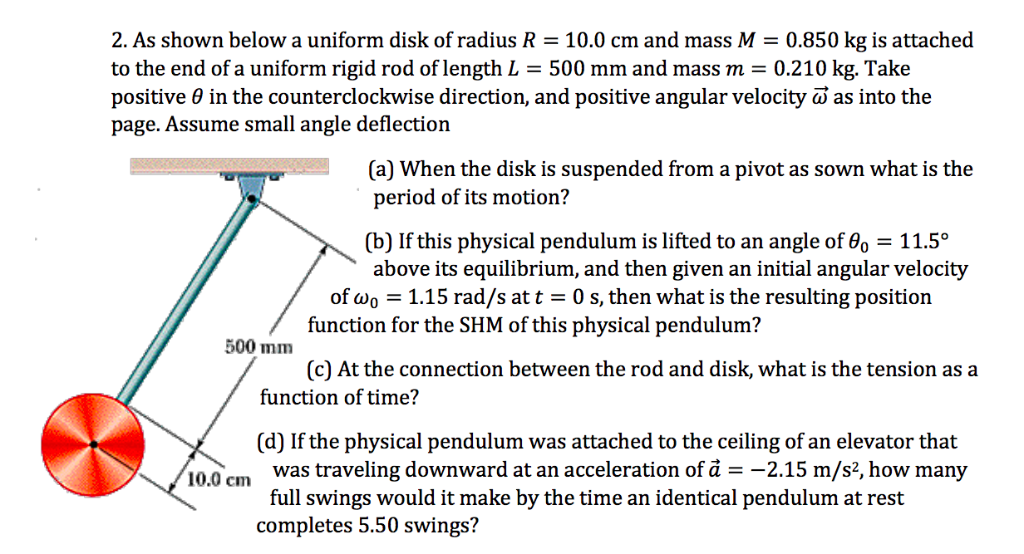2. As shown below a uniform disk of radius R 10.0 cm and mass M 0.850 kg is attached to the end of a uniform rigid rod of length L = 500 mm and mass m = 0.210 kg. Take positive θ in the counterclockwise direction, and positive angular velocity ω as into the page. Assume small angle deflection (a) When the disk is suspended from a pivot as sown what is the period of its motion? (b) If this...